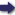(London :  Kegan Paul, Trench, Trübner & Co.,  1910.)

 Tools

## Search this bookPrev Page 76 Next``` 76 ALBERUNTS INDIA. Criticisms on Brahma¬ gupta's method. by the divisor kept in memory) by the diameter of the earth. The product is the distance between the earth's centre and the end of the shadow. Subtract there¬ from the true distance of the moon and multiply the remainder by the diameter of the earth. Divide the product by the true distance of the shadow's end. The quotient is the diameter of the shadow in the sphere of the moon. Further, we suppose the true distance of the moon to be LS, and FN is a part of the lunar sphere, the radius of which is LS. Since we have found LM as determined by the minutes of the sine, it stands in the same relation to CD, this being the double sinus totus, as MS, measured in minutes of the sine, to XZ, measured in minutes of the sine." Here I suppose Brahmagupta wished to reduce LM, the true distance of the shadow's end, to yojanas, which is done by multiplying it by the yojctnas of the diameter of the earth, and by dividing the product by the double sinus totus. The mentioning of this division has fallen out in the manuscript; for without it the multiplication of the corrected distance of the shadow's end by the diameter of the earth is perfectly superfluous, and in no way required by the computation. Further : " If the number of yojctncts of LM is known, LS, which is the true distance, must also be reduced to yojctnas, for the purpose that MS should be determined by the same measure. The measure of the diameter of te shadow which is thus found represents yojanas. Further, Brahmagupta says : " Then multiply the shadow which has been found by the sinus totus, and divide the product by the true distance of the moon. The quotient represents the minutes of the shadow which we wanted to find." However, if the shadow which he has found were determined by yojctnas, he ought to have multiplied it by the double sinus totus, and to have divided the pro¬ duct by the yojctnas of the diameter' of the earth, in ```Prev Page 76 Next